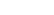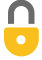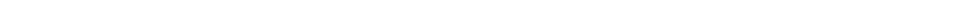Problem 12
Q

# Write the following as full (decimal) numbers without prefixes on the units:

1. $286.6 \textrm{ mm}$,
2. $85 \; \mu \textrm{V}$,
3. $760 \textrm{ mg}$,
4. $62.1 \textrm{ ps}$,
5. $22.5 \textrm{ nm}$,
6. $2.50 \textrm{ gigavolts}$.A
a) $0.2886 \textrm{ m}$
b) $0.000085 \textrm{ V}$
c) $0.76 \textrm{ g}$
d) \$0.0000000000621 s
e) 0.0000000225 m
f) 2500000000 VIn order to watch this solution you need to have a subscription.VIDEO TRANSCRIPT

This is Giancoli Answers with Mr. Dychko. The prefix milli means multiply by 10 to the negative 3 and so we have this 286.6 times 10 to the minus 3 well, times 10 to the minus 3 means move the decimal place that we currently have three spaces to the left. So we have once, twice, three times to the left puts the decimal place here just to the left of the 2 and we write in a placeholder zero 0.2866 meters. Micro means multiply by 10 to the minus 6 and so we have 85 with the decimal point assumed to be there, we'll go six to the left, once, twice, three, four, five, six times puts it there 0.000085 volts. Milli—three to the left— and starts here once, twice, three times and we don't repeat the zero here because strictly speaking, this zero is not significant there's nothing to indicate that it's an actual measured zero rather it's just there as a placeholder to put the 7 in the hundreds place and the 6 in the tens place and if there had been a decimal point here then we would assume the zero was a measured number and significant, in other words, and we would have written 0.760 but in this case, it's not significant and we will not write it because there's no need to write it except to suggest that it is significant which it is not. OK. So we have 0.76 grams. Pico is 10 to the minus 12 and so we take this decimal place and move it twelve times to the left and so it started here just between the 2 and the 1 so that's once to the left twice, three, four, five, six, seven, eight, nine, ten, eleven, twelve points to the left and that puts it there. And "nano" is times 10 to the minus 9 so decimal point began here between the 2 and the 5 and then go nine to the left and you get this number. And giga is times 10 to the positive 9 and that means when exponent is positive, you move the decimal place to the right that many times, same number of times as the exponent. So we have this decimal point going nine times to the right. And there we go.

Giancoli Answers, including solutions and videos, is copyright © 2009-2023 Shaun Dychko, Vancouver, BC, Canada. Giancoli Answers is not affiliated with the textbook publisher. Book covers, titles, and author names appear for reference purposes only and are the property of their respective owners. Giancoli Answers is your best source for the 7th and 6th edition Giancoli physics solutions.